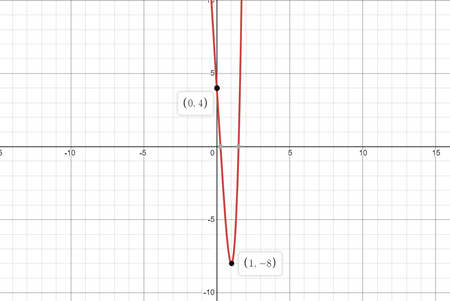# Consider the function f (x) = 4 x^4 - 16 x + 4. (a) Find all relative extrema. (b) Find the point...

## Question:

Consider the function {eq}\displaystyle f (x) = 4 x^4 - 16 x + 4 {/eq}.

(a) Find all relative extrema.

(b) Find the point of inflection.

(c) Use the graphing utility to graph the function.

## Relative Extrema and Inflection Points:

For a function {eq}\displaystyle f(x) {/eq}, the points of extrma are given by the solutions to the equation {eq}\displaystyle f'(x)=0 {/eq}.

These points are also called as critical points.

The point at which the function changes its concavity is called the inflection point.

The inflection points are given by the solutions of the equation {eq}\displaystyle f''(x)=0 {/eq}.

Given function {eq}\displaystyle f(x)=4 x^4 - 16 x + 4 {/eq}.

Now,

a.

Points of extrema are given by:

{eq}\displaystyle \begin{align} f'(x)&=0\\ 16x^3-16&=0\\ x^3-1&=0\\ x&=1. \end{align} {/eq}

b.

Inflection points of the function are givne by:

{eq}\displaystyle \begin{align} f''(x)&=0\\ 48x^2&=0\\ x&=0 \end{align} {/eq}

{eq}\displaystyle \Rightarrow {/eq} Inflection point is found at {eq}\displaystyle x=0 {/eq}.

c.

Graph of the function: